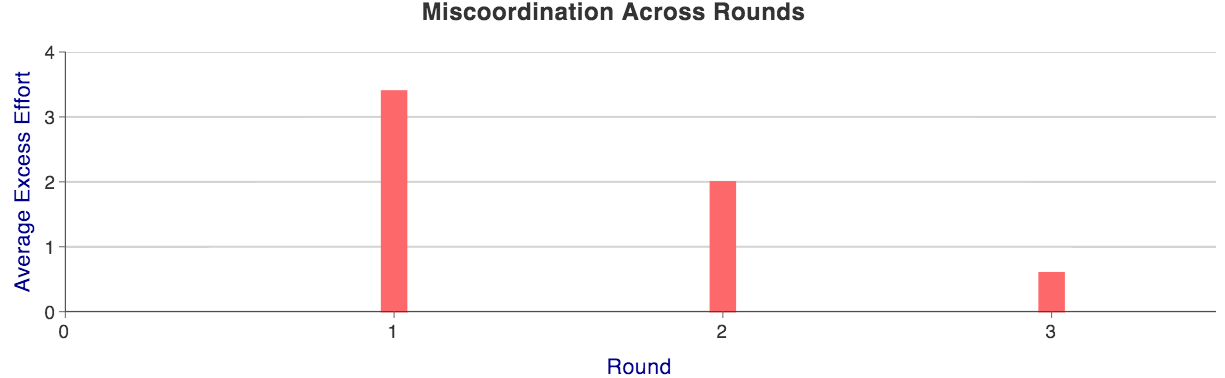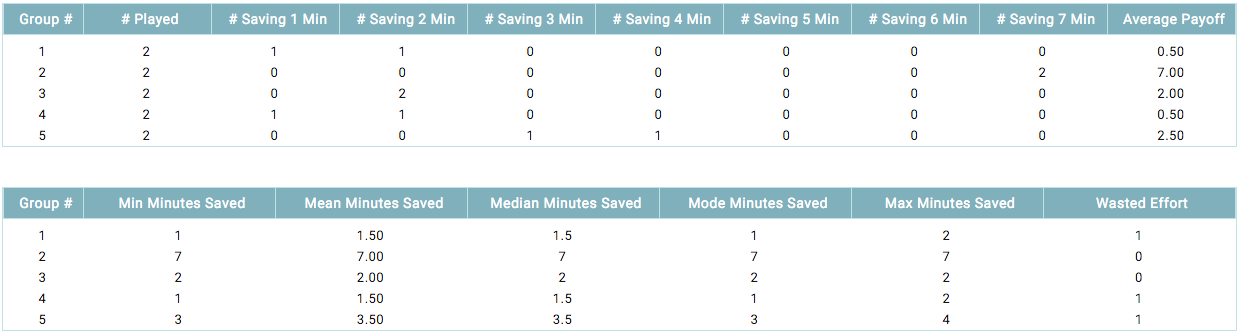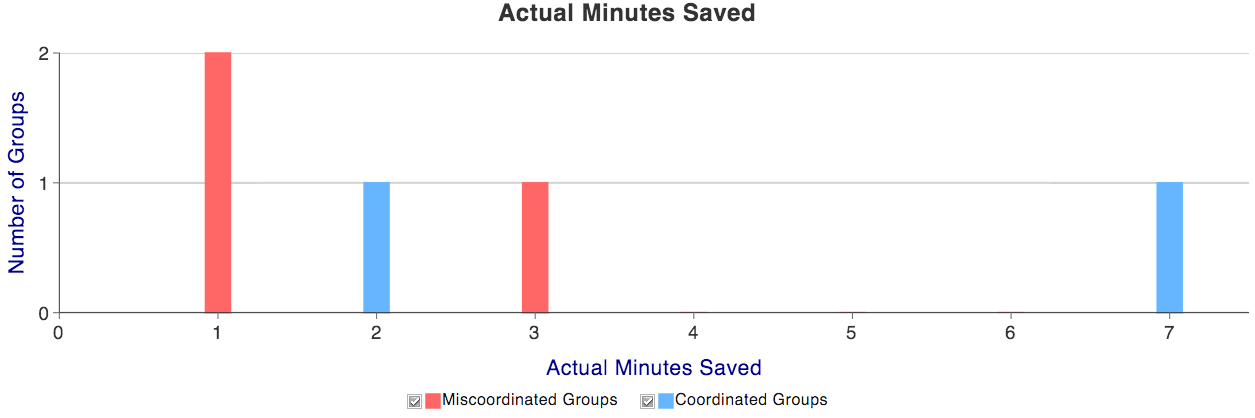MobLab
Guides
Aa

# Minimum Effort

## Game Description

Based on the classic coordination game (Van Huyck, Battalio and Beil, 19901): equilibria can be Pareto ranked, but actions leading to more efficient equilibria have the highest costs of miscoordination. This results in strategic uncertainty, with equilibrium “riskiness” increasing in its Pareto ranking.

Students play airline employees readying a plane for takeoff. Each group member receives a bonus proportional to the number of minutes saved. Each round, each chooses how many minutes to save, and pays a cost proportional to her choice. Choices are revealed, and the actual time saved is the smallest choice amongst all group members (i.e., minimum effort).

### Learning objective 1: (Mis)coordination in games with multiple equilibria

Players may fail to coordinate on a unique payoff-dominant equilibrium (i.e., all players agree on the most preferred equilibrium) if choosing the associated action exposes the player strategic risk.

### Learning objective 2: Coordination in games with multiple equilibria

Repeat interaction likely reduces miscoordination. While coordination on a unique payoff-dominant equilibrium may be attractive, play may converge to less efficient equilibria if choosing the action in payoff-dominant equilibrium exposes the player to strategic risk. Both communication and target setting can increase the likelihood of the payoff dominant equilibrium.

## Brief Instructions

The default parameters will work for a vast majority of instructors. Group Size is 6, and each group member chooses an effort level between Minimum Effort Level=1 and Maximum Effort Level=7. An individual pays a \$1 per unit of effort she chooses (Cost of Effort), and each receives a bonus per minute actually saved (Payoff of Effort) of \$2 (where minutes actually saved equals the minimum effort chosen by a member of the group).

In any pure-strategy equilibrium, each group member chooses the same level of effort, and coordinating on any effort level is an equilibrium. Each choosing Maximum Effort Level is the payoff-dominant equilibrium. Of course, choosing Maximum Effort Level exposes the player to the worst possible outcome (if a group member chooses 1). Maximin play (choosing the action with the largest payoff in the worst possible outcome) results a choosing Minimum Effort Level.

In a single-round game (Minimum Rounds=1 and Ending Probability (%)=100), you will likely see significant miscoordination, with the smallest effort chosen within each group (i.e., actual minutes saved) at or near Maximum Effort Level and many within each group choosing effort at or near Maximum Effort Level.

## Key Treatment Variations

Repetition with group composition fixed (i.e., Minimum Rounds>1) will see a reduction in miscoordination. However, most groups will come to coordinate on lower rather higher levels of effort as last period’s minimum often serves as a current period anchor.

Three options will likely increase coordination on higher levels of effort. The first is to allow group members to communicate (enabling Chat). Second, you can have an effort-level target announced to each group member. To do so, change Target Effort from 0 to the desired target. Finally, smaller groups will have more minutes saved (on average). Decreasing Groups Size works in two ways. Splitting any group will likely result in one subgroup having a larger minimum than the original group, and students might respond with an increase in effort.

## Results

The results screen shows per-group (mis)coordination in a particular round as well as the trend across rounds. To switch between rounds, and the across-round summary, use the dropdown menu at the upper-left of the results screen.Figure 1: Across-round summaries

We present two graphs summarizing outcomes across rounds (Figure 1). The first presents excess effort averaged across all groups for each round. You should expect improved coordination as round progress, and thus a decreasing trend in excess effort. The second chart displays minutes saved averaged across all groups for each round. It is common to see little movement in the average minimum effort as rounds progress.Figure 2: Within-round summary tables

For each round, we present two tables and a graph. The first table (Figure 2) shows the distribution of choices for each group. The final column, Average Payoff, summarizes the cost of miscoordination. Per-person equilibrium payoffs range from 1 to 7 (with the default parameters), and it is not uncommon to see negative average payoffs in Round 1. The second table (also Figure 2) summarizes the per-group choice distributions, displaying for each group minimum, maximum, mean, median and mode choices. The first column calculates the minimum effort level chosen in each group, and the last column adds up all wasted effort.Figure 3: Within-round outcome distribtuion

The graph we present for each round (Figure 3) shows for each actual number of minutes saved (horizontal axis) the number groups with that outcome (vertical axis). Those outcomes where all group members made the same choice are displayed in blue, whereas groups without perfect coordination are displayed in red. By clicking on the checkbox next to the outcome type, you can surpress its display in the graph and thus focus on either coordinated or miscoordinated groups.

1. Van Huyck, John B. and Raymond C. Battalio and Richard O. Beil (1990). “Tacit Coordination Games, Strategic Uncertainty, and Coordination Failure.” The American Economic Review, 84(1): 234–248.